# How to use ISERROR function in Excel

The tutorial looks at the practical uses of the Excel ISERROR function and shows how to test different formulas for errors.

When you write a formula that Excel does not understand or cannot calculate, it draws your attention to the problem by showing an error message. The ISERROR function can help you catch errors and provide an alternative when an error is found.

## ISERROR function in Excel

The Excel ISERROR function catches all kinds of errors, including #CALC!, #DIV/0!, #N/A, #NAME?, #NUM!, #NULL!, #REF!, #VALUE!, and #SPILL!. The result is a Boolean value: TRUE if an error is detected, FALSE otherwise.

The function is available in all versions of Excel 2000 through 2021 and Excel 365.

The syntax of the ISERROR function is as simple as this:

ISERROR(value)

Where value is the cell value or formula to be checked for errors.

## Excel ISERROR formula

To create an ISERROR formula in its simplest form, supply a reference to the cell that you want to test for errors. For example:

`=ISERROR(A2)`

In case any error is found, you'll get TRUE. If there is no error in the tested cell, you'll get FALSE: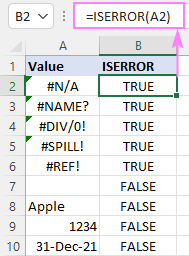## IF ISERROR formula in Excel

To return a custom message or perform a different calculation when an error occurs, use ISERROR together with the IF function. The generic formula looks as follows:

IF(ISERROR(formula(…), text_or_calculation_if_error, formula())

Translated into a human language, it says: if the main formula results in an error, display the specified text or run another calculation, otherwise return a normal result of the formula.

In the image below, dividing the total by the quantity generates a couple of errors in the Price column: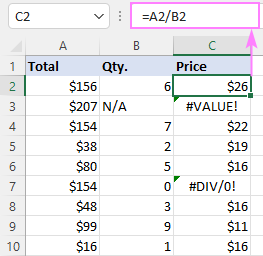To replace all different error codes with a custom text, you can use the following IF ISERROR formula:

`=IF(ISERROR(A2/B2), "Unknown", A2/B2)`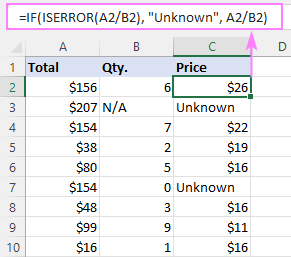In Excel 2007 and later versions, the same result can be achieved with the help of the inbuilt IFERROR function:

`=IFERROR(A2/B2, "Unknown")`

It should be noted that the IFERROR formula runs a little faster because it performs the A2/B2 calculation just once. Whereas IF ISERROR calculates it twice - first to see if it generates an error and then again if the test is FALSE.

## IF ISERROR VLOOKUP formula

Using ISERROR with VLOOKUP is, in fact, a particular case of the IF ISERROR formula discussed above. When the VLOOKUP function cannot find the lookup value or fails for any other reason, you display a custom text message by using this syntax:

IF(ISERROR(VLOOKUP(…)), "custom_text", VLOOKUP(…))

For this example, let's pull the times from the lookup table (D3:E10) to the main table (A3:B15). If the lookup value (participant's name) does not exist in the lookup table, we'll return "Not qualified".

`=IF(ISERROR(VLOOKUP(A3, \$D\$3:\$E\$10, 2, FALSE)), "Not qualified", VLOOKUP(A3, \$D\$3:\$E\$10, 2, FALSE))`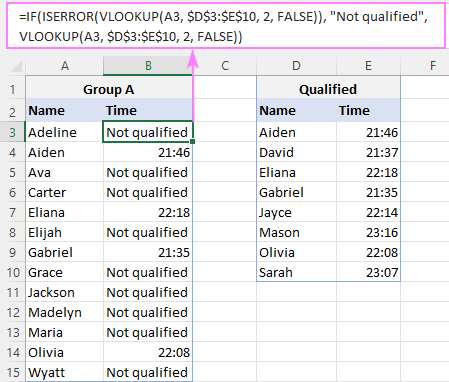Tip. If you want to display a custom text only when a lookup value is not found (#N/A error) ignoring other errors, then use the IFNA VLOOKUP formula in Excel 2013 and later or IF ISNA VLOOKUP in older versions.

## IF ISERROR INDEX MATCH formula

When performing a lookup with the help of the INDEX MATCH combination (or INDEX XMATCH formula in Excel 365), you can trap and handle any possible errors by using the same technique - the ISERROR function checks for errors and IF displays the specified text when any error occurs.

IF(ISERROR(INDEX (return_column, MATCH (lookup_value, lookup_column, 0)))), "custom_text", INDEX (return_column, MATCH (lookup_value, lookup_column, 0)))

Suppose the lookup table has times in the first column. As VLOOKUP is unable to look to its left, we use the INDEX MATCH formula to pull the times from column D:

`=INDEX(\$D\$3:\$D\$10, MATCH(A3, \$E\$3:\$E\$10, 0))`

And then, you nest it in the above-mentioned generic formula to replace the caught errors with any text you want:

`=IF(ISERROR(INDEX(\$D\$3:\$D\$10, MATCH(A3, \$E\$3:\$E\$10, 0))), "Not qualified", INDEX(\$D\$3:\$D\$10, MATCH(A3, \$E\$3:\$E\$10, 0)))`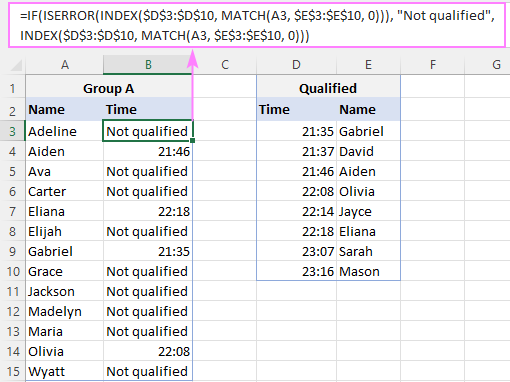Note. As with the IF ISERROR VLOOKUP formula, it makes more sense to trap only #N/A errors and do not disguise potential problems with the formula itself. For this, wrap your INDEX MATH formula in IFNA in Excel 2013 and higher or IF ISNA in earlier versions.

## IF ISERROR Yes/No formula

In all the previous examples, IF ISERROR returned the result of the main formula if it isn't an error. However, it can also work in a different way - return something if error and something else if no error.

IF(ISERROR(formula(…)), "text_if_error", "text_if_no_error")

In our sample dataset, suppose you are not interested in the exact times, you just want to know which participants from group A are qualified and which are not. To do this, use the MATCH function to compare the name in column A against the list of the qualified participants in column D, and then serve the results to ISERROR. If the name is not available in column D (MATCH returns an error), get the IF function to display "No" or "Not qualified". If the name appears in column D (no error), return "Yes" or "Qualified".

`=IF(ISERROR(MATCH(A3, \$D\$3:\$D\$10, 0)), "No", "Yes" )`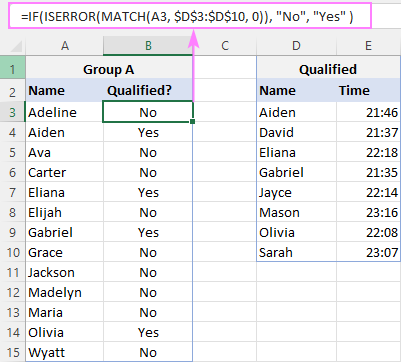## How to count the number of errors

To get the number of errors in a certain column, you need to check a range, not just one cell. For this, "feed" the target range to ISERROR and coerce the returned Boolean values into 1's and 0's using the double unary operator (--). The SUM or SUMPRODUCT function can add up the numbers and deliver the final result.

For example:

`=SUM(--ISERROR(C2:C10))`

Please note, this works as a regular formula only in Excel 365 and Excel 2021, which support dynamic arrays. In Excel 2019 and earlier, you need to press Ctrl + Shift + Enter to create an array formula (don't type curly brackets manually, that won't work!):

`{=SUM(--ISERROR(C2:C10))}`

Alternatively, you can use the SUMPRODUCT function that handles arrays natively, so the formula can be completed with the usual Enter key in all versions:

`=SUMPRODUCT(--ISERROR(C2:C10))`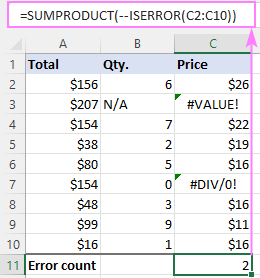## Difference between ISERROR and IFERROR in Excel

Both the ISERROR and IFERROR functions are used to trap and handle errors in Excel. The difference is as follows:

• In its pure form, ISERROR just tests if the value is an error or not. It's available in all Excel versions.
• The IFERROR function is designed to suppress or disguise errors - when an error is found, it returns another value that you specify. It is available in Excel 2007 and higher.

At first sight, IFERROR looks like a shorthand alternative to the IF ISERROR formula. On a closer look, however, you can notice the difference:

• IFERROR allows you to specify only value_if_error. If there is no error, it always returns the result of the tested value/formula.
• IF ISERROR provides more flexibility and lets you handle both situations - what should happen if an error and what if no error.

To better illustrate the point, consider these formulas:

`=IFERROR(A1, "Calculation error")`

`=IF(ISERROR(A1), "Calculation error", A1)`

These two formulas are equivalent - both check a formula-driven value in A1 and return "Calculation error" if it's an error, otherwise - return the value.

But what if you want to perform some calculation if the value in A1 is not an error? The IFERROR function is unable to do that. In case of IF ISERROR, just type the desired calculation in the last argument. For example:

`=IF(ISERROR(A1), "Calculation error", A1*2)`

As you see, this longer variation of the IFERROR formula, which is often considered outdated, can still be useful :)